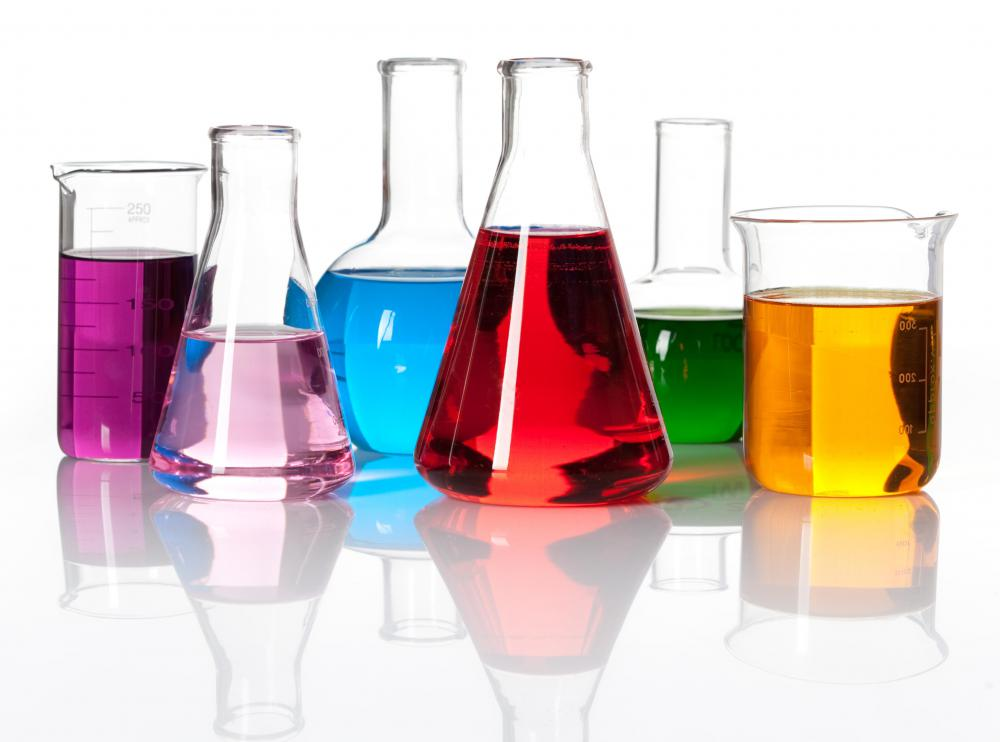# What Is Percent Composition? (with picture)

C.H. Seman
C.H. SemanSubstances with various compositions.

In chemistry, the percent composition of a component in a compound is the percent of the total mass or volume of the compound because of that specific component. It is a simple way of expressing concentration in analytical chemistry. Percent composition may refer to either weight percent or volume percent, although weight percent is the most commonly used.

Although percent composition may refer to composition by mass or volume, the calculations for both are similar. Weight percent, often denoted "wt%" in calculation shorthand, is calculated by dividing the mass of a solute or component by the mass of the total solution or compound and multiplying the result by 100. Volume percent, denoted by "vol%" in calculation shorthand, is calculated by dividing the volume of a solute or component by the entire volume of the total solution or compound and multiplying the result by 100. When reporting this in laboratory work, it is important to make the distinction between weight percent and volume percent to avoid ambiguity.

A common application for weight composition calculations can be found in the analysis of elements in a molecule. For example, to calculate the percent composition of oxygen in ethanol, one would first determine the mass of oxygen in 1 mole of ethanol, divide that number by the total mass of 1 mole of ethanol, and then multiply the result by 100. Ethanol(CH3CH2OH) has a molar mass of approximately 46.069 grams per mole, and oxygen has a molar mass of 15.999 grams per mole. The percent composition of oxygen in ethanol would be (15.999/46.069)*100, or 34.73%.

In gas chromatography, the weight composition of a substance can be determined by dividing the substance's peak value by the sum of all the peak values in the chromatograph read out. This is a particularly useful technique when multiple unknown components may exist in the compound. As with all gas chromatography readings, however, it is important for the chemist to consider the effects of the carrier gas on the components, the response factors of the different components, and the general shape of the peaks before drawing any definite conclusions about percent composition.

Percent composition can be related to other methods of measurement in analytical chemistry and may be used to calculate measurements such as molarity. For example, if a solution of hydrochloric acid in water is said to have a density of 1.188 grams per milliliter and the percent composition of hydrochloric acid is 37 percent by weight, then the molarity of the solution can be shown to be 12.1. This is accomplished by using the percent composition to determine the number of moles of hydrochloric acid in 1 liter of the solution.

## You might also Like## Discuss this Article

Forgot password?
###### Register:
•Substances with various compositions.